Valency What is valency? In order to write chemical formulae we need to understand how elements combine with each other to form molecules or ionic compounds. Ionic compounds are substances made from the combination of charged ( positive and negative) particles. Valency refers to the ability of an atom or a group of chemically bonded atoms to form chemical bonds with other atoms or groups of atoms. The valency of an element is determined by the number of outer shell (valence) electrons. The valency of polyatomic ions (such as SO42- ) is the charge on the ion. Let's not over complicate this and go straight into the periodic table and how we can tell the valency of an element.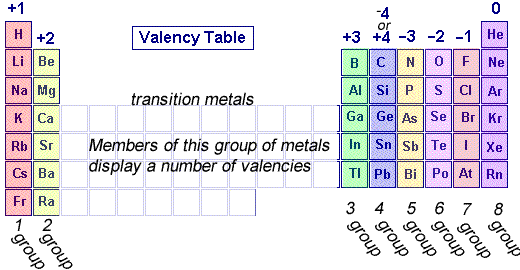The periodic table, shown on the left, can tell us a great deal about the valency of elements. Elements are placed in groups (columns) in the periodic table according to the number of valence electrons, so naturally the position of the element in the periodic table should give us an idea of its valency. All elements in group 1 have 1 valence electron so they have a valency of +1 as they will tend to give up 1 electron. This is the same for group 2 which will give up two electrons and group 3 which will give up 3 electron . Group 5 elements, however, have 5 valence electrons and will tend to take 3 electrons and so have a valency of -3. Group 6 elements, have 6 valence electrons and will tend to take 2 electrons and have a valency of -2. Group 7 elements have 7 valence electrons and will tend to take 1 electron and have a valency of -1. Group 8 elements do not react and so have a valency of 0 . Valencies come in really handy when we are looking to write formulae of compounds formed from metal and non-metal atoms. For example take the reaction between sodium and chlorine atoms. Compounds formed between metals and non-metals will be treated differently. First we will deal with metal, non-metal interactions. Lithium has a valency of +1 and nitrogen has a valency of -3 Combine the atoms of nitrogen and lithium to give the formula of lithium nitride. When writing the formula the idea is to create a neutral compound by using as few atoms from each element as possible. Follow this very simple method. Step 1 Write down the two atoms and their valency always putting the atom with the positive valency on the left.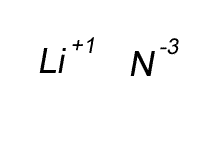Step 2 Write the valency (without the sign) for each atom as a subscript.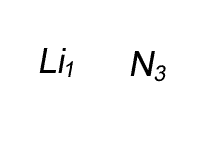Step 3 Swap the subscripts and divide by the smallest number only if the larger number is divisible by the smaller number.Step 4 Remove any subscript that is "1"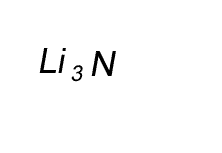Lets look at another example by writing the formula of the compound formed between oxygen and aluminium (aluminium oxide) Step 1 Write down the two atoms and their valency always putting the atom with the positive valency on the left.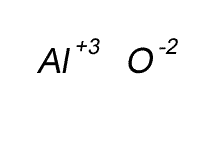Step 2 Write the valency (without the sign) for each atom as a subscript.Step 3 Swap the subscripts and divide by the smallest number only if the larger number is divisible by the smaller number.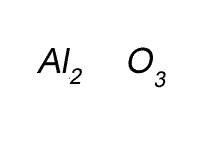Step 4 Remove any subscript that is "1"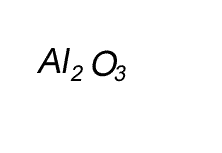Write the formula for the compound formed between calcium and carbon called calcium carbide. Step 1 Write down the two atoms and their valency always putting the atom with the positive valency on the left.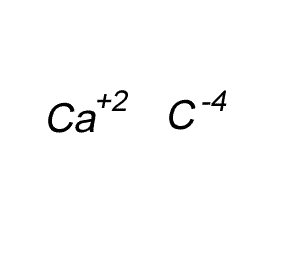Step 2 Write the valency (without the sign) for each atom as a subscript.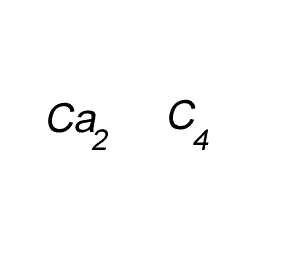Step 3 Swap the subscripts and divide by the smallest number only if the larger number is divisible by the smaller number.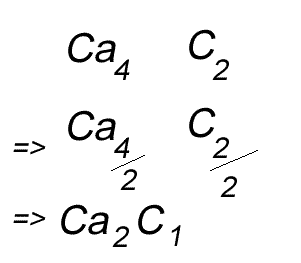Step 4 Remove any subscript that is "1"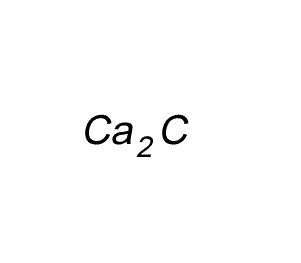Try some for yourself. Write the formula for the compound formed between: a) calcium and nitrogen (calcium nitride),b) boron and oxygen (boron oxide) c) fluorine and aluminium (aluminium fluoride) d) tin and nitrogen (tin nitride) e) oxygen and lithium (lithium oxide) f) phosphorus and calcium (calcium phosphide) Solutions Stephen was asked to write the formula of the compound formed when boron and oxygen atoms combine. He wrote the formula as "O3B2". Is this correct? Explain Solution